# Tag Archives: No G&J reference

## Maximum Likelihood Ranking

This is another (hopefully the last, we’re getting close to the end) “private communication” problem where I couldn’t find a paper to use, so I had to work through it myself, with mixed success.  Let’s see how I do.

The problem: Maximum Likelihood Ranking.  This is problem MS11 in the appendix.

The description: Suppose we have an nxn matrix A with integer values.  Further, let aij + aji = 0.  We’re also given a positive integer B.  Can we do “simultaneous row and column permutations” to A to create another matrix (G&J call it B, but I’ll call it C to avoid confusion), such that: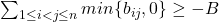?

The description (my interpretation): Here’s what I think that means.  You have a directed  graph with just one edge in each direction between any 2 vertices, represented as an adjacency matrix  A positive entry in the matrix is the cost to traverse the edge, and a negative entry in the matrix means you’re traversing the edge backwards.  Can I permute the rows and columns of the matrix so that the “backwards” edges in all positions where i < j sum to -B at most?”

Example: Here is a graph: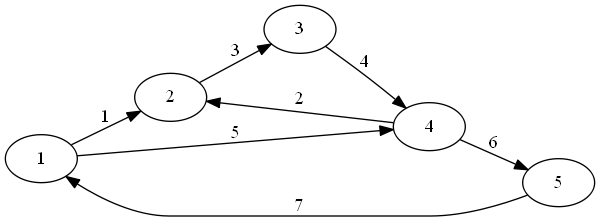Here is its matrix: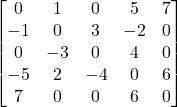The negative numbers in the upper right triangle is what we are looking at to compare against our B- in this case it’s -9.

I’m pretty sure a “simultaneous row and column permutation” means you do the same permutation to the rows and to the columns.  So if the above matrix was {1,2,3,4,5}, here is {2,3,4,5,1}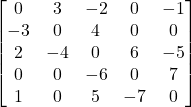The negatives in the upper right triangle here sum to -8 (so it’s better).  It’s worth noticing that we have two cycles in the graph (2->3->4->2 and 1->4->5->1), and that any permutation will have to have a “backwards” ordering for one of the cycles.  So at least 1 edge in each cycle will have its negative value counted in our total, so this graph will be at least -7.

(Partial) Reduction: As I said, this is a “private communication” reduction, our only hint is that we are supposed to use Feedback Arc Set.  What Feedback Arc Set asks for is a set of edges that is in each directed cycle.

So, let’s try this:  Given a directed graph G=(V,E) that’s our Feedback Arc Set graph, represent it as an adjacency matrix such that aij = 1 if edge (i,j) is in E, and aij = -1 if (j,i) is in E.  Our B will be the K from the problem.

My intuition is that each cycle will have to contribute 1 edge (at least) that is negative in our upper triangle.  So if there are K edges that cover all cycles, we would get the B cost from those edges in our problem.  We want the edge in the feedback arc set to be last in the permutation order.  For example, here is a directed graph: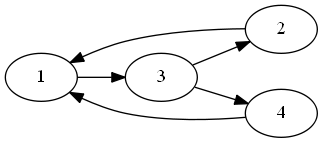Here is its graph with the regular ordering: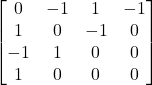..for a cost of -3.  But we can solve the FBS instance with just 1 edge from 1-3.  So here is the matrix will all edges going forward except for that one (3,2,4,1 ordering):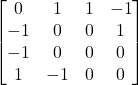..for a cost of -1 (because there is just 1 edge in the Feedback Arc Set)

I’d like to have my reduction be someting like “put all edges in the Feedback Arc Set in the matrix in order where they go backwards”.  But I don’t know what to do with graphs like this, where the Feedback Arc Set itself is a cycle, so you can’t do that: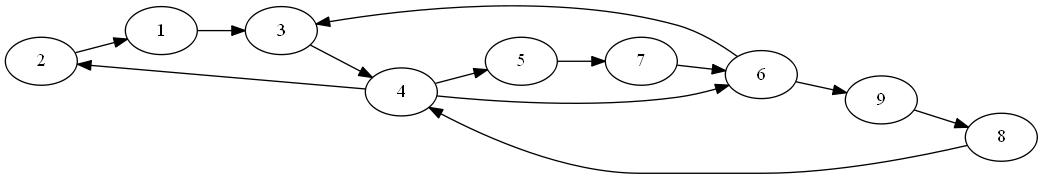{(3,4), (4,6), (6,3)} is the Feedback Arc Set here, but because it is itself a cycle, there is no permutation of vertices that will put all of the edges in the upper right.  I think this is a consequence of there being 4 cycles in the graph, but the Feedback Arc Set having just 3 edges.  But I’m not sure, and so we need to have something more in the reduction.

Difficulty: ??  It doesn’t look terribly hard, but I’m not sure what to do next.